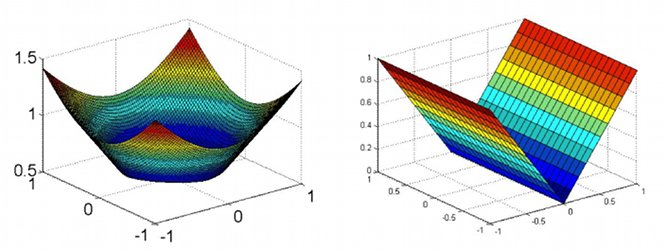Numerical Solution of the Monge-Ampere Equation

Associated People:

Adam Oberman (Simon Fraser University)

Brittany Froese (Simon Fraser University)

Associated Sites:
PIMS Simon Fraser University

The elliptic Monge-Ampere equation is a fully nonlinear Partial Differential Equation that originated in geometric surface theory and has been applied in dynamic meteorology, elasticity, geometric optics, image processing and image registration. Solutions can be singular, in which case standard numerical approaches fail.

Prof. Oberman and his colleague have developed a finite difference solver for the Monge-Ampere equation which converges even for singular solutions. Regularity results are used to select a priori between a stable, provably convergent monotone discretization and an accurate finite difference discretization in different regions of the computational domain. The resulting nonlinear equations are then solved by Newton's method, thus allowing both singular solutions to be computed and regular solutions to be computed more accurately.References
• https://wiki.sfu.ca/personal/aoberman/index.php/Main_Page